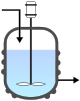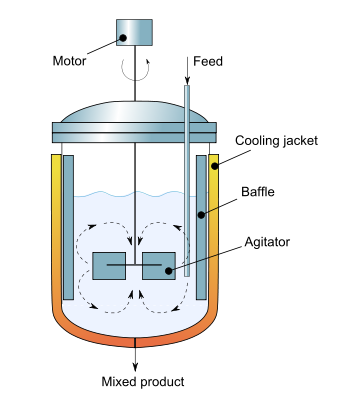# Continuous stirred-tank reactor

﻿
Continuous stirred-tank reactorCSTR symbol

The continuous stirred-tank reactor (CSTR), also known as vat- or backmix reactor, is a common ideal reactor type in chemical engineering. A CSTR often refers to a model used to estimate the key unit operation variables when using a continuous[†] agitated-tank reactor to reach a specified output. (See Chemical reactors.) The mathematical model works for all fluids: liquids, gases, and slurries.

The behavior of a CSTR is often approximated or modeled by that of a Continuous Ideally Stirred-Tank Reactor (CISTR). All calculations performed with CISTRs assume perfect mixing. In a perfectly mixed reactor, the output composition is identical to composition of the material inside the reactor, which is a function of residence time and rate of reaction. If the residence time is 5-10 times the mixing time, this approximation is valid for engineering purposes. The CISTR model is often used to simplify engineering calculations and can be used to describe research reactors. In practice it can only be approached, in particular in industrial size reactors.

Assume:

• perfect or ideal mixing, as stated above

Integral mass balance on number of moles Ni of species i in a reactor of volume V. [accumulation] = [in] − [out] + [generation]

1.$\frac{dN_i}{dt} = F_{io} - F_i + V \nu_i r_i$Cross-sectional diagram of Continuous stirred-tank reactor

where Fio is the molar flow rate inlet of species i, Fi the molar flow rate outlet, and νi stoichiometric coefficient. The reaction rate, r, is generally dependent on the reactant concentration and the rate constant (k). The rate constant can be determined by using a known empirical reaction rates that is adjusted for temperature using the Arrhenius temperature dependence. Generally, as the temperature increases so does the rate at which the reaction occurs. Residence time, τ, is the average amount of time a discrete quantity of reagent spends inside the tank.

Assume:

• constant density (valid for most liquids; valid for gases only if there is no net change in the number of moles or drastic temperature change)
• isothermal conditions, or constant temperature (k is constant)
• single, irreversible reactionA = -1)
• first-order reaction (r = kCA)

A → products

NA = CA V (where CA is the concentration of species A, V is the volume of the reactor, NA is the number of moles of species A)

2.$C_A = \frac {C_{Ao}}{1 + k \tau }$ 

The values of the variables, outlet concentration and residence time, in Equation 2 are major design criteria.

To model systems that do not obey the assumptions of constant temperature and a single reaction, additional dependent variables must be considered. If the system is considered to be in unsteady-state, a differential equation or a system of coupled differential equations must be solved.

CSTR's are known to be one of the systems which exhibit complex behavior such as steady-state multiplicity, limit cycles and chaos.

3. Non-ideal CSTR

The actual reaction volume will be affected by the non-uniform mixing (short-circuiting) or existence of dead zone areas. Temperature effect will also cause significant effect on the reaction rate.

Wikimedia Foundation. 2010.

### Look at other dictionaries:

• Continuous reactor — Continuous reactors (alternatively referred to as flow reactors) carry material as a flowing stream. Reactants are continuously fed into the reactor and emerge as continuous stream of product. Continuous reactors are used for a wide variety of… …   Wikipedia

• Chemical reactor — In chemical engineering, chemical reactors are vessels designed to contain chemical reactions. The design of a chemical reactor deals with multiple aspects of chemical engineering. Chemical engineers design reactors to maximize net present value… …   Wikipedia

• Plug flow reactor model — The plug flow reactor (PFR) model is used to describe chemical reactions in continuous, flowing systems. The PFR model is used to predict the behaviour of chemical reactors, so that key reactor variables, such as the dimensions of the reactor,… …   Wikipedia

• Chemostat — Stirred bioreactor operated as a chemostat, with a continuous inflow (the feed) and outflow (the effluent). The rate of medium flow is controlled to keep the culture volume constant. A chemostat (from Chemical environment is static) is a… …   Wikipedia

• Mass balance — A mass balance (also called a material balance) is an application of conservation of mass to the analysis of physical systems. By accounting for material entering and leaving a system, mass flows can be identified which might have been unknown,… …   Wikipedia

• Residence Time Distribution — The residence time distribution (RTD) of a chemical reactor is a probability distribution function that describes the amount of time a fluid elements could spend inside the reactor. Chemical engineers use the RTD to characterize the mixing and… …   Wikipedia

• Membrane bioreactor — (MBR) is the combination of a membrane process like microfiltration or ultrafiltration with a suspended growth bioreactor, and is now widely used for municipal and industrial wastewater treatment with plant sizes up to 80,000 population… …   Wikipedia

• Biochemical engineering — is a branch of chemical engineering or biological engineering that mainly deals with the design and construction of unit processes that involve biological organisms or molecules. Biochemical engineering is often taught as a supplementary option… …   Wikipedia

• Anaerobic digester types — The following is a partial list of different types of anaerobic digesters. These processes and systems harness anaerobic digestion for purposes such as sewage treatment and biogas generation.Examples include: *Anaerobic activated sludge process… …   Wikipedia

• Réacteur (chimie) — Pour les articles homonymes, voir Réacteur. En chimie, un réacteur est une enceinte ou récipient apte à la réalisation et l optimisation de réactions chimiques et généralement de procédés de transformation de la matière (génie des procédés). Le… …   Wikipédia en Français